#Incompressible Flow
The module implements the basic fluid objects necessary to represent incompressible, two-phase flow. To solve these flow equations, the module offers a standard two-point flux-approximation (TPFA) method for pressure and explicit and implicit transport solvers using single-point upstream mobility weighting, which can be combined in sequential solution procedures.

## Tutorials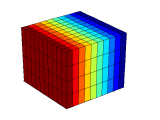### Basic flow solver

The example explains how to use the standard two-point pressure solver to solve the single-phase pressure equation for a flow driven by Dirichlet and Neumann boundary conditions.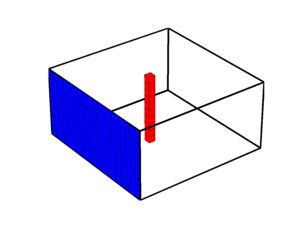### Source and boundary conditions

This example shows how to set up a single-phase flow driven by a combination of source and boundary conditions and is a continuation of the basic flow-solver tutorial.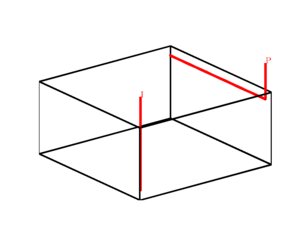### Wells models

This examples explains how to extend the single-phase model with a standard Peaceman model to represent a vertical injector and a horizontal producer.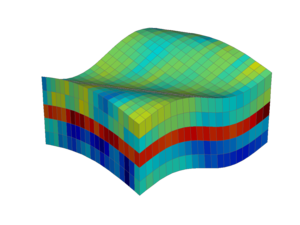### Corner-point grids

The example shows how to generate a simple grid on the industry-standard corner-point format, generate a geostatistical realization of a layered permeability field, and solve the resulting single-phase flow problem.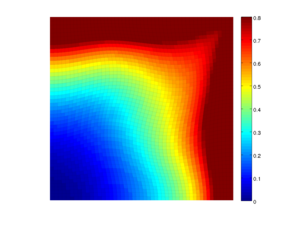### Time-of-flight intro

Time-of-flight represents the time it takes an imaginary particle released at an inflow boundary or at a perforation of an injector to reach a given point in the reservoir. This defines natural time-lines in the reservoir, which indicate how heterogeneity affects flow patterns in the reservoir.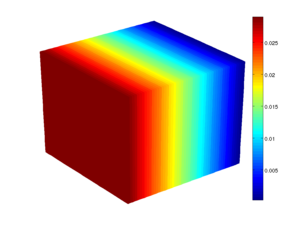### Million-cell model

The example shows how to solve models with multi-million cells by using C-accelerated geometry computation and a highly efficient algebraic multigrid method (AGMG) to solve the single-phase pressure equation.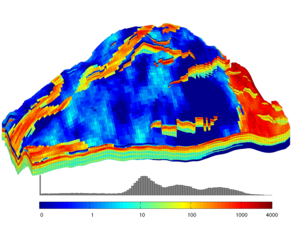### A SAIGUP model

The purpose of the SAIGUP project was to investigate the influence of geological features on the oil recovery from shallow-marine reservoirs. The example explains how to set up a single-phase flow simulation on one of the geostatistical models in the project. The model has faults and inactive cells, but no pinchout, and is an example of a realistic reservoir model.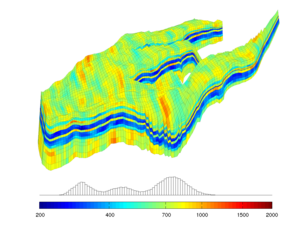### The Norne field

The example shows how to set up a simplified simulation model using the reservoir geometry and petrophysical parameters from the simulation model of the Norne field. This is an example of a real grid model that has degenerate cell geometries and non-neighbouring connections arising from a large number of faults, eroded layers, pinch-outs, and inactive cells.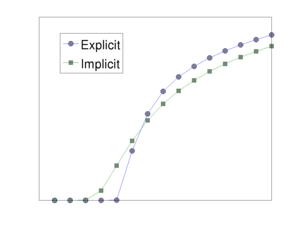### Basic two-phase solvers

We explain how to solve a two-phase flow problem using the explicit and implicit transport solvers. The setup is the same as in the simple well example with a vertical injector and a horizontal producer.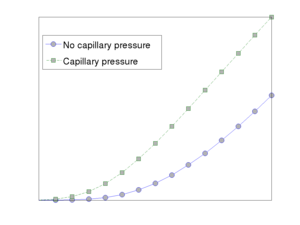### Including capillary pressure

The example compares and contrasts the displacement profile and production curves for a standard quater-five spot problem with and without capillary forces.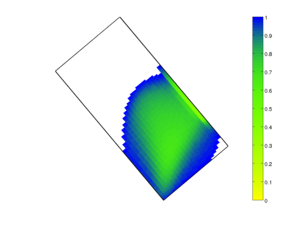### Gravity migration

The example studies how a light fluid (CO2) injected at the bottom of an inclined and sealing aquifer will migrate to the top of the formation, where it will be structurally trapped.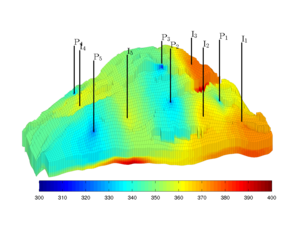### SAIGUP: waterflooding

The example computes a simplified waterflooding scenario using the reservoir geometry and the petrophysical properties from a synthetic SAIGUP model. The model has 78 720 active cells and to speed up the computation, we use the AGMG multigrid solver when computing pressure.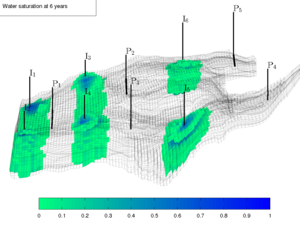### Norne: waterflooding

The example computes a simplified waterflooding scenario using the reservoir geometry and the petrophysical properties from the Norne field model.

## Description

MRST's fluid representation is a structure of function handles which compute fluid properties (e.g., density or viscosity), fluid phase saturations, and relative-permeability and capillary-pressure curves. This representation supports generic implementation of derived quantities such as mobilities or flux functions for a wide range of fluid models. The `incomp` module offers the following fluid-object constructors:

• `initSingleFluid` - a single-phase fluid with prescribed density and viscosity. Input parameters: density, viscosity
• `initSimpleFluid` - a simplified Corey-type two-phase fluid with relative permeabilities of the form krw(S)=S^n, and kro(S)=(1-S)^m. Input parameters: densities, viscosities, and [n,m].
• `initSimpleFluidPc` - same as above, but with a linear capillary pressure Pc(S) = scale*(1-S).
• `initSimpleFluidJfunc` - same relative permeability model as in `initSimpleFluid`, but with a Leverett-J function that scales the capillary pressure by petrophysical parameters. Additional input: rock structure containing valid poro and perm fields and surface tension
• `initCoreyFluid` - the full Corey model with residual saturations and end-point scaling. Input parameters: densities, viscosities, residual saturations, [n,m], and end-point scaling [kwm,kom]

To solve the resulting single-phase and two-phase incompressible fluid equations that can be modelled with the above fluid objects, the module offers three solvers:

• `incompTPFA` - a pressure solver that implements the two-point flux-approximation method. The solver relies on half-transmissibilities computed using the `computeTrans` function from the core module
• `explicitTransport` - a saturation solver that implements a single-point, upwind-mobility-weighting scheme with explicit time stepping
• `implicitTransport` - a saturation solver that implements a single-point, upwind-mobility-weighting scheme with implicit time stepping

The three solvers should, at least in principle, be applicable to any kind of incompressible fluid model that follows the same convention as the fluid objects already implemented in the module. It is therefore not difficult to extend the functionality of the module to contain other relative-permeability models, more phases, etc.

The incomp module was originally implemented as part of MRST's core functionality, but has been moved to a separate module from release 2015a and onward.

## Literature

Published December 8, 2016